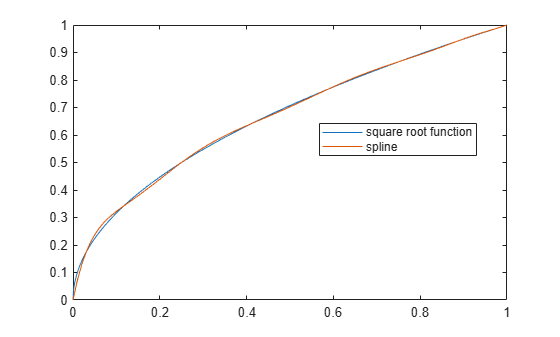# chbpnt

Chebyshev-Demko points

## Syntax

```tau = chbpnt(t,k) chbpnt(t,k,tol) [tau,sp] = chbpnt(...) ```

## Description

`tau = chbpnt(t,k) `are the extreme sites of the Chebyshev spline of order `k` with knot sequence `t`. These are particularly good sites at which to interpolate data by splines of order `k` with knot sequence `t` because the resulting interpolant is often quite close to the best uniform approximation from that spline space to the function whose values at `tau` are being interpolated.

`chbpnt(t,k,tol) ` also specifies the tolerance `tol` to be used in the iterative process that constructs the Chebyshev spline. This process is terminated when the relative difference between the absolutely largest and the absolutely smallest local extremum of the spline is smaller than `tol`. The default value for `tol` is `.001`.

`[tau,sp] = chbpnt(...) ` also returns, in `sp`, the Chebyshev spline.

## Examples

collapse all

Create a knot sequence of ten zeros and ten ones using the `augknt` function.

```k = 10; t = augknt([0,1],k);```

Create a vector of interpolation sites by calculating the Chebyshev-Demko points for the Chebyshev spline of order 10 with knot sequence `t`.

`sites = chbpnt(t,k);`

Create a vector of interpolation points by evaluating the function `sqrt` at the Chebyshev-Demko points. Use the function `spapi` to interpolate through the evaluated points with a tenth-order spline from the knot sequence `t`. The knots in `t` indicate that the spline fit consists of a single polynomial with boundaries at 0 and 1.

```interpolationPoints = sqrt(sites); sp = spapi(t,sites,interpolationPoints)```
```sp = struct with fields: form: 'B-' knots: [0 0 0 0 0 0 0 0 0 0 1 1 1 1 1 1 1 1 1 1] coefs: [-1.7088e-16 0.8842 -0.7101 2.4484 -1.1869 1.9678 0.3331 0.9940 0.9349 1] number: 10 order: 10 dim: 1 ```

The structure `sp` contains the properties of the spline. The `coefs` field of `sp` is a vector of coefficients for the polynomial that approximates the square root function in the interval `[0,1]`.

Compare `sp` and the square root function by displaying them in the same plot.

```x = linspace(0,1,1000); plot(x,sqrt(x)) hold on fnplt(sp,'-',0.75) legend(["square root function" "spline"],Location="best")```The plot shows that the spline closely follows the square root function.

## Algorithms

The Chebyshev spline for the given knot sequence and order is constructed iteratively, using the Remez algorithm, using as initial guess the spline that takes alternately the values 1 and −1 at the sequence `aveknt(t,k)`. The example Construct Chebyshev Spline gives a detailed discussion of one version of the process as applied to a particular example.